SLVS515D December   2004  – November 2015

PRODUCTION DATA.

1. Features
2. Applications
3. Description
4. Revision History
5. Pin Configuration and Functions
6. Specifications
7. Parameter Measurement Information
8. Detailed Description
1. 8.1 Overview
2. 8.2 Functional Block Diagram
3. 8.3 Feature Description
4. 8.4 Device Functional Modes
9. Application and Implementation
1. 9.1 Application Information
2. 9.2 Typical Application
10. 10Power Supply Recommendations
11. 11Layout
12. 12Device and Documentation Support
13. 13Mechanical, Packaging, and Orderable Information

• PWP|28
• RHB|32
• PWP|28
• RHB|32

## 6 Specifications

### 6.1 Absolute Maximum Ratings

over operating free-air temperature range (unless otherwise noted)(1)
MIN MAX UNIT
Input voltage(2) VCC –0.3 6 V
V(BLANK), V(DCPRG), V(SCLK), V(XLAT), V(SIN), V(GSCLK), V(IREF) –0.3 VCC +0.3 V
Output voltage V(SOUT), V(XERR) –0.3 VCC +0.3 V
V(OUT0) to V(OUT15) –0.3 18 V
Output current (dc) 130 mA
EEPROM program range V(VPRG) –0.3 24 V
EEPROM write cycles 50
Storage temperature, Tstg –55 150 °C
(1) Stresses beyond those listed under may cause permanent damage to the device. These are stress ratings only, which do not imply functional operation of the device at these or any other conditions beyond those indicated under . Exposure to absolute-maximum-rated conditions for extended periods may affect device reliability.
(2) All voltage values are with respect to network ground terminal.

### 6.2 ESD Ratings

VALUE UNIT
V(ESD) Electrostatic discharge Human-body model (HBM), per ANSI/ESDA/JEDEC JS-001(1) ±2000 V
Charged-device model (CDM), per JEDEC specification JESD22-C101(2) ±500
(1) JEDEC document JEP155 states that 500-V HBM allows safe manufacturing with a standard ESD control process.
(2) JEDEC document JEP157 states that 250-V CDM allows safe manufacturing with a standard ESD control process.

### 6.3 Recommended Operating Conditions

MIN NOM MAX UNIT
DC CHARACTERISTICS
VCC Supply Voltage 3 5.5 V
V O Voltage applied to output (OUT0–OUT15) 17 V
VIH High-level input voltage 0.8 VCC VCC V
VIL Low-level input voltage GND 0.2 VCC V
IOH High-level output current VCC = 5 V at SOUT –1 mA
IOL Low-level output current VCC = 5 V at SOUT, XERR 1 mA
IOLC Constant output current OUT0 to OUT15, VCC < 3.6 V 60 mA
OUT0 to OUT15, VCC > 3.6 V 120 mA
V(VPRG) EEPROM program voltage 20 22 23 V
TA Operating free-air temperature range -40 85 °C
AC CHARACTERISTICS
VCC = 3 V to 5.5 V, TA = –40°C to 85°C (unless otherwise noted)
f(SCLK) Data shift clock frequency SCLK 30 MHz
f(GSCLK) Grayscale clock frequency GSCLK 30 MHz
twh0/twl0 SCLK pulse duration SCLK = H/L (see Figure 11) 16 ns
twh1/twl1 GSCLK pulse duration GSCLK = H/L (see Figure 11) 16 ns
twh2 XLAT pulse duration XLAT = H (see Figure 11) 20 ns
twh3 BLANK pulse duration BLANK = H (see Figure 11) 20 ns
tsu0 Setup time SIN to SCLK ↑(1) (see Figure 11) 5 ns
tsu1 SCLK ↓ to XLAT ↑ (see Figure 11) 10 ns
tsu2 VPRG ↑ ↓ to SCLK ↑ (see Figure 11) 10 ns
tsu3 VPRG ↑ ↓XLAT ↑ (see Figure 11) 10 ns
tsu4 BLANK ↓ to GSCLK ↑ (see Figure 11) 10 ns
tsu5 XLAT ↑ to GSCLK ↑ (see Figure 11) 30 ns
tsu6 VPRG ↑ to DCPRG ↑ (see Figure 16) 1 ms
th0 Hold Time SCLK ↑ to SIN (see Figure 11) 3 ns
th1 XLAT ↓ to SCLK ↑ (see Figure 11) 10 ns
th2 SCLK ↑ to VPRG ↑ ↓ (see Figure 11) 10 ns
th3 XLAT ↓ to VPRG ↑ ↓ (see Figure 11) 10 ns
th4 GSCLK ↑ to BLANK ↑ (see Figure 11) 10 ns
th5 DCPRG ↓ to VPRG ↓ (see Figure 11) 1 ms
tprog Programming time for EEPROM (see Figure 16) 20 ms
(1) ↑ and ↓ indicates a rising edge, and a falling edge respectively.

### 6.4 Thermal Information

THERMAL METRIC(1) TLC5940 UNIT
PWP (HTSSOP) RHB (VQFN)
28 PINS 32 PINS
RθJA Junction-to-ambient thermal resistance 36.7 34.3 °C/W
RθJC(top) Junction-to-case (top) thermal resistance 18.9 36.8 °C/W
RθJB Junction-to-board thermal resistance 15.9 8.5 °C/W
ψJT Junction-to-top characterization parameter 0.6 0.3 °C/W
ψJB Junction-to-board characterization parameter 15.8 8.7 °C/W
RθJC(bot) Junction-to-case (bottom) thermal resistance 2.3 1.6 °C/W

### 6.5 Electrical Characteristics

VCC = 3 V to 5.5 V, TA = –40°C to 85°C (unless otherwise noted)
PARAMETER TEST CONDITIONS MIN TYP MAX UNIT
VOH High-level output voltage IOH = -1 mA, SOUT VCC –0.5 V
VOL Low-level output voltage IOL = 1 mA, SOUT 0.5 V
II Input current VI = VCC or GND; BLANK, DCPRG, GSCLK, SCLK, SIN, XLAT –1 1 μA
VI = GND; VPRG –1 1
VI = VCC; VPRG 50
VI = 22 V; VPRG; DCPRG = VCC 4 10 mA
ICC Supply current No data transfer, all output OFF,
VO = 1 V, R(IREF) = 10 kΩ
0.9 6 mA
No data transfer, all output OFF,
VO = 1 V, R(IREF) = 1.3 kΩ
5.2 12
Data transfer 30MHz, all output ON,
VO = 1 V, R(IREF) = 1.3 kΩ
16 25
Data transfer 30MHz, all output ON,
VO = 1 V, R(IREF) = 640 Ω
30 60
IO(LC) Constant sink current (see Figure 10) All output ON, VO = 1 V, R(IREF) = 640 Ω 54 61 69 mA
Ilkg Leakage output current All output OFF, VO = 15 V, R(IREF) = 640 Ω,
OUT0 to OUT15
0.1 μA
ΔIO(LC0) Constant sink current error (see Figure 10) All output ON, VO = 1 V, R(IREF) = 640 Ω,
OUT0 to OUT15, –20°C to 85°C
±1% ±4%
All output ON, VO = 1V, R(IREF) = 640 Ω,
OUT0 to OUT15(1)
±1% ±8%
All output ON, VO = 1V, R(IREF) = 320 Ω,
OUT0 to OUT15, –20°C to 85°C
±1% ±6%
All output ON, VO = 1V, R(IREF) = 320 Ω,
VCC = 4.5 V to 5.5 V, OUT0 to OUT15(1)
±1% ±8%
ΔIO(LC1) Constant sink current error (see Figure 10) Device to device, Averaged current from OUT0 to OUT15, R(IREF) = 1920 Ω (20 mA)(2) –2%
+0.4%
±4%
ΔIO(LC2) Constant sink current error (see Figure 10) Device to device, Averaged current from OUT0 to OUT15, R(IREF) = 480 Ω (80 mA)(2) –2.7%
+2%
±4%
ΔIO(LC3) Line regulation (see Figure 10) All output ON, VO = 1V, R(IREF) = 640 Ω
OUT0 to OUT15, VCC = 3 V to 5.5 V(3)
±1 ±4 %/V
All output ON, VO = 1V, R(IREF) = 320 Ω ,
OUT0 to OUT15, VCC = 3 V to 5.5 V(3)
±1 ±6 %/V
ΔIO(LC4) Load regulation (see Figure 10) All output ON, VO = 1 V to 3 V, R(IREF) = 640 Ω,
OUT0 to OUT15(4)
±2 ±6 %/V
All output ON, VO = 1 V to 3 V, R(IREF) = 320 Ω,
OUT0 to OUT15(4)
±2 ±8 %/V
T(TEF) Thermal error flag threshold Junction temperature(5) 150 170 °C
V(LED) LED open detection threshold 0.3 0.4 V
V(IREF) Reference voltage
output
R(IREF) = 640 Ω 1.20 1.24 1.28 V
(1) The deviation of each output from the average of OUT0-15 constant current. It is calculated by Equation 1 in Test Parameter Equations.
(2) The deviation of average of OUT1-15 constant current from the ideal constant-current value. It is calculated by Equation 2 in Test Parameter Equations. The ideal current is calculated by Equation 3 in Test Parameter Equations.
(3) The line regulation is calculated by Equation 4 in Test Parameter Equations.
(4) The load regulation is calculated by Equation 5 in Test Parameter Equations.
(5) Not tested. Specified by design

### 6.6 Switching Characteristics

VCC = 3V to 5.5V, TA = -40°C to 85°C (unless otherwise noted)
PARAMETER TEST CONDITIONS MIN TYP MAX UNIT
tr0 Rise time SOUT 16 ns
tr1 OUTn, VCC = 5 V, TA = 60°C, DCn = 3 Fh 10 30
tf0 Fall time SOUT 16 ns
tf1 OUTn, VCC = 5 V, TA = 60°C, DCn = 3 Fh 10 30
tpd0 Propagation delay time SCLK to SOUT (see Figure 11) 30 ns
tpd1 BLANK to OUT0 60 ns
tpd2 OUTn to XERR (see Figure 11) 1000 ns
tpd3 GSCLK to OUT0 (see Figure 11) 60 ns
tpd4 XLAT to IOUT (dot correction) (see Figure 11) 60 ns
tpd5 DCPRG to OUT0 (see Figure 11) 30 ns
td Output delay time OUTn to OUT(n+1) (see Figure 11) 20 30 ns
ton-err Output on-time error touton– Tgsclk (see Figure 11), GSn = 01h, GSCLK = 11 MHz 10 –50 –90 ns

### 6.7 Typical CharacteristicsFigure 1. Reference Resistor vs Output Current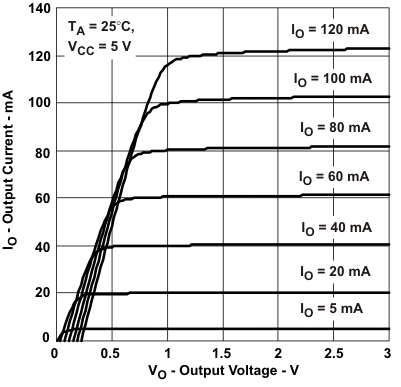Figure 3. Output Current vs Output Voltage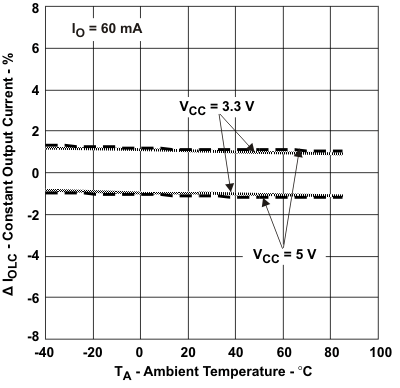Figure 5. Constant Output Current, ΔIOLC
vs Ambient Temperature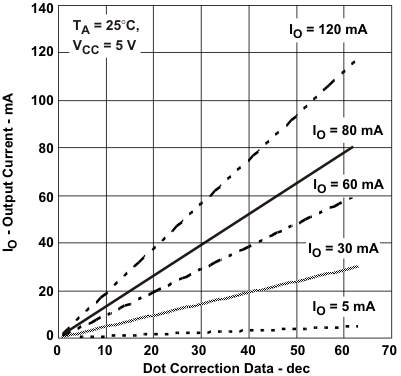Figure 7. Output Current
vs DOT Correction Linearity (ABS Value)Figure 2. Power Dissipation Rate vs Free-Air Temperature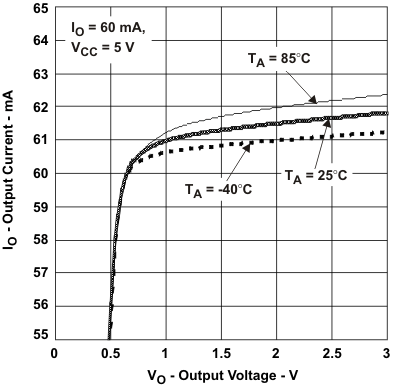Figure 4. Output Current vs Output Voltage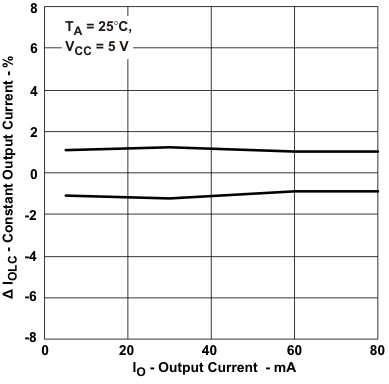Figure 6. Constant Output Current, ΔIOLC
vs Output Current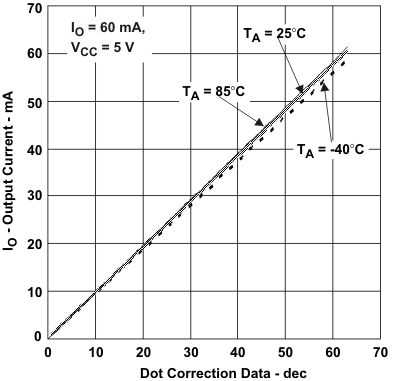Figure 8. Output Current
vs DOT Correction Linearity (ABS Value)